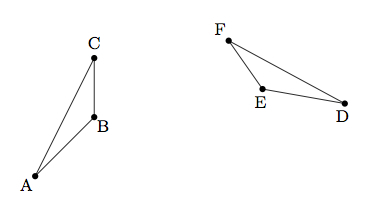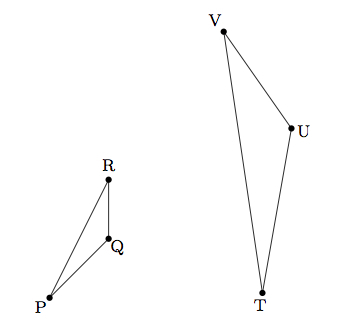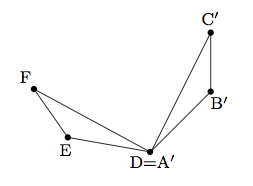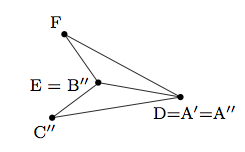# Congruent and Similar Triangles

Alignments to Content Standards: G-SRT.A.2

1. In triangles $ABC$ and $DEF$ below $m(\angle A) = m(\angle D)$, $m(\angle B) = m(\angle E)$ and $|\overline{AB}| = |\overline{DE}|$.Find a sequence of translations, rotations, and reflections which maps $\triangle ABC$ to $\triangle DEF$.

2. After working on problem (a), Melissa says
Since $m(\angle A) = m(\angle D)$ and $m(\angle B) = m(\angle E)$ then I also know that $m(\angle C) = m(\angle F)$. So these triangles share all three angles and this is enough to know that they are congruent. I don't need to be told that $|\overline{AB}| = |\overline{DE}|$.
1. Is Melissa correct that $m(\angle C) = m(\angle F)$? Explain.
2. Is she right that two triangles sharing three pairs of congruent angles are always congruent? Explain.
3. Below are two triangles which share three congruent angles: $m(\angle P) = m(\angle T)$, $m(\angle Q) = m(\angle U)$, $m(\angle R) = m(\angle V)$:Show that, after applying a suitable dilation, $\triangle PQR$ is congruent to $\triangle TUV$.

## IM Commentary

The goal of this task is to understand similarity as a natural extension of congruence. Two geometric shapes are congruent when there is a sequence of translations, reflections, and rotations which maps one shape to the other. Two shapes are similar when there is a sequence of translations, reflections, rotations, and dilations mapping one shape to the other. The normal criterion for checking similarity of triangles, AA, is investigated here from the perspective of students who have just finished working on congruence.

All of the congruence criteria (SSS, SAS, ASA) involve three corresponding parts. This makes sense because a triangle is determined by three points and so three pieces of information would be expected in order to determine a triangular shape. Interestingly enough, however, not any three pieces of information are sufficient: for example SSA does not allow us to conclude congruence except with additional hypotheses (though it is very restrictive). The other natural candidate, AAA, is also insufficient and Melissa's reasoning in part (b) is perhaps suggestive of why: specifying two congruent pairs of angles for two triangles guarantees three congruent angles so in fact, though AAA looks like three pieces of information, we are actually only being given two independent pieces of information.

## Solution

1. There are many ways to show the congruence between $\triangle ABC$ and $\triangle DEF$. One method is to first translate $A$ to $D$. This is shown below and the translated triangle is denoted $A^\prime B^\prime C^\prime$.We know that $|AB| = |A^\prime B^\prime| = |DE|$ since rigid motions preserve distances between points. Next we would like to move $B^\prime$ to $E$ which we can do via a rotation through $\angle B^\prime DE$. This is shown below with the rotated triangle marked $A^{\prime \prime}B^{\prime \prime}C^{\prime \prime}$.We know that $|DE| = |AB| = |A^\prime B^\prime| = |A^{\prime \prime}B^{\prime \prime}|$ since rigid motions preserve distances between points. Since $\overrightarrow{A^{\prime \prime}B^{\prime \prime}} = \overrightarrow{DE}$ and $A^{\prime \prime} = D$ we conclude that $E = B^{\prime \prime}$ as shown in the picture above. Finally we can apply a reflection about $\overleftrightarrow{DE}$. This reflection maps $\overrightarrow{DC^{\prime \prime}}$ to $\overrightarrow{DF}$ because $m(\angle EDF) = m(\angle BAC) = m(\angle B^{\prime \prime}A^{\prime \prime} C^{\prime \prime})$. Similarly, the reflection maps $\overrightarrow{EC^{\prime \prime}}$ to $\overrightarrow{EF}$ because $m(\angle DEF) = m(\angle ABC) = m(\angle A^{\prime \prime}B^{\prime \prime}C^{\prime \prime})$. This means that reflection over $\overleftrightarrow{DE}$ maps $C^{\prime \prime}$ to $F$ and shows the congruence between $\triangle ABC$ and $\triangle DEF$.

1. Melissa is correct that $m(\angle C) = m(\angle F)$ because $$m(\angle C) = 180 - m(\angle A) - m(\angle B) = 180 - m(\angle D) - m(\angle E) = m(\angle F).$$
2. Two triangles sharing three pairs of congruent angles are similar but not necessarily congruent. For example, an equilateral triangle with side lengths 1 is not congrunt to an equilateral triangle with side lengths 2. They are similar, however, with a scale factor of 2.
2. If we dilate $\triangle PQR$ with center $P$ by a scale factor of $\frac{|TU|}{|PQ|}$, we know that $P$ does not move and the image $Q^\prime$ of $Q$ will satisfy $$|PQ^\prime| = \frac{|TU|}{|PQ|}.$$ In particular, we have $|PQ^\prime| = |TU|$. This is pictured below:Dilations preserve lines through the center of the dilation so $Q^\prime$ lies on $\overleftrightarrow{PQ}$. Dilations also preserve angles so $m(\angle P^\prime Q^\prime R^\prime) = m(\angle PQR)$ and $m(\angle P^\prime R^\prime Q^\prime) = m(\angle PRQ)$. This means that $\triangle P^\prime Q^\prime R^\prime$ is congruent to $\triangle TUV$ by ASA. Concretely in terms of transformations, we can translate $P$ to $T$ and then a rotation as in part (a) completes the congruence as the two triangles $P^\prime Q^\prime R^\prime$ and $TUV$ have the same orientation and a final reflection is not needed.